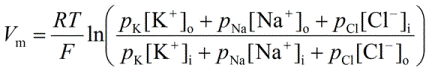PhysiologyWeb  Home  |  FAQ  |  About  |  Contact  |   - Sign InTweetPhysiology Test Question
In a typical cell in which K+, Na+, and Cl contribute to the value of the resting membrane potential (Vm), the following equation can be used to calculate the value of Vm.
 (A)   The Nernst equation(B)   The Goldman-Hodgkin-Katz (GHK) equation